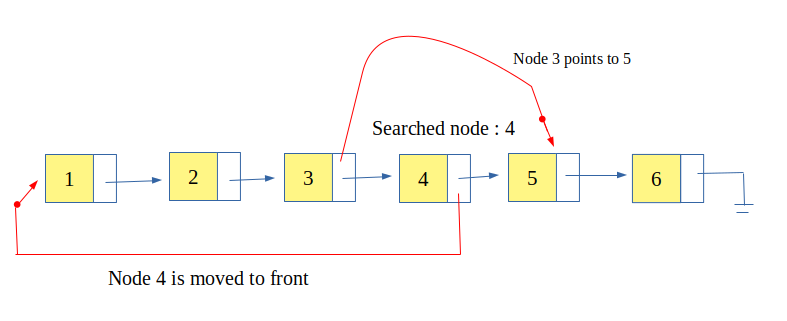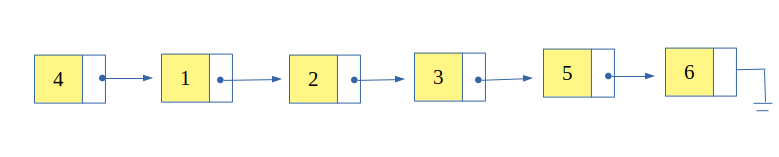Related Articles

# Self Organizing List : Move to Front Method

• Difficulty Level : Easy
• Last Updated : 28 Dec, 2017

Self Organizing list is a list that re-organizes or re-arranges itself for better performance. In a simple list, an item to be searched is looked for in a sequential manner which gives the time complexity of O(n). But in real scenario not all the items are searched frequently and most of the time only few items are searched multiple times.

So, a self organizing list uses this property (also known as locality of reference) that brings the most frequent used items at the head of the list. This increases the probability of finding the item at the start of the list and those elements which are rarely used are pushed to the back of the list.

Attention reader! Don’t stop learning now. Get hold of all the important DSA concepts with the DSA Self Paced Course at a student-friendly price and become industry ready.  To complete your preparation from learning a language to DS Algo and many more,  please refer Complete Interview Preparation Course.

In case you wish to attend live classes with experts, please refer DSA Live Classes for Working Professionals and Competitive Programming Live for Students.

In Move to Front Method, the recently searched item is moved to the front of the list. So, this method is quite easy to implement but it also moves in-frequent searched items to front. This moving of in-frequent searched items to the front is a big disadvantage of this method because it affects the access time.Examples:

```Input :   list : 1, 2, 3, 4, 5, 6
searched: 4
Output :  list : 4, 1, 2, 3, 5, 6

Input :   list : 4, 1, 2, 3, 5, 6
searched : 2
Output :  list : 2, 4, 1, 3, 5, 6
```
 `// CPP Program to implement self-organizing list``// using move to front method``#include ``using` `namespace` `std;`` ` `// structure for self organizing list``struct` `self_list {``    ``int` `value;``    ``struct` `self_list* next;``};`` ` `// head and rear pointing to start and end of list resp.``self_list *head = NULL, *rear = NULL;`` ` `// function to insert an element``void` `insert_self_list(``int` `number)``{``    ``// creating a node``    ``self_list* temp = (self_list*)``malloc``(``sizeof``(self_list));`` ` `    ``// assigning value to the created node;``    ``temp->value = number;``    ``temp->next = NULL;`` ` `    ``// first element of list``    ``if` `(head == NULL)``        ``head = rear = temp;`` ` `    ``// rest elements of list``    ``else` `{``        ``rear->next = temp;``        ``rear = temp;``    ``}``}`` ` `// function to search the key in list``// and re-arrange self-organizing list``bool` `search_self_list(``int` `key)``{``    ``// pointer to current node``    ``self_list* current = head;`` ` `    ``// pointer to previous node``    ``self_list* prev = NULL;`` ` `    ``// searching for the key``    ``while` `(current != NULL) {`` ` `        ``// if key found``        ``if` `(current->value == key) {`` ` `            ``// if key is not the first element``            ``if` `(prev != NULL) {`` ` `                ``/* re-arranging the elements */``                ``prev->next = current->next;``                ``current->next = head;``                ``head = current;``            ``}``            ``return` `true``;``        ``}``        ``prev = current;``        ``current = current->next;``    ``}`` ` `    ``// key not found``    ``return` `false``;``}`` ` `// function to display the list``void` `display()``{``    ``if` `(head == NULL) {``        ``cout << ``"List is empty"` `<< endl;``        ``return``;``    ``}`` ` `    ``// temporary pointer pointing to head``    ``self_list* temp = head;``    ``cout << ``"List: "``;`` ` `    ``// sequentially displaying nodes``    ``while` `(temp != NULL) {``        ``cout << temp->value;``        ``if` `(temp->next != NULL)``            ``cout << ``" --> "``;`` ` `        ``// incrementing node pointer.``        ``temp = temp->next;``    ``}``    ``cout << endl  << endl;``}`` ` `// Driver Code``int` `main()``{``    ``/* inserting five values */``    ``insert_self_list(1);``    ``insert_self_list(2);``    ``insert_self_list(3);``    ``insert_self_list(4);``    ``insert_self_list(5);`` ` `    ``// Display the list``    ``display();`` ` `    ``// search 4 and if found then re-arrange``    ``if` `(search_self_list(4))``        ``cout << ``"Searched: 4"` `<< endl;``    ``else``        ``cout << ``"Not Found: 4"` `<< endl;`` ` `    ``// Display the list``    ``display();`` ` `    ``// search 2 and if found then re-arrange``    ``if` `(search_self_list(2))``        ``cout << ``"Searched: 2"` `<< endl;``    ``else``        ``cout << ``"Not Found: 2"` `<< endl;``    ``display();`` ` `    ``return` `0;``}`

Output:

```List: 1 --> 2 --> 3 --> 4 --> 5

Searched: 4
List: 4 --> 1 --> 2 --> 3 --> 5

Searched: 2
List: 2 --> 4 --> 1 --> 3 --> 5
```

My Personal Notes arrow_drop_up